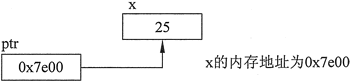## 指针变量是什么，C++指针变量详解

• 内容
• 评论
• 相关

int *ptr;

int* ptr;

```//This program stores the address of a variable in a pointer.
#include <iostream>
using namespace std;
int main()
{
int x = 25; // int variable
int *ptr; // Pointer variable, can point to an int
ptr = &x; // Store the address of x in ptr
cout << "The value in x is " << x << endl;
cout << "The address of x is " << ptr << endl;
return 0;
}```

The value in x is 25
The address of x is 0x7e00

ptr = &x;0条评论